## Section30.4Electric Field for Cylindrical Symmetry

### Subsection30.4.1Cylindrical Symmetry

Figure 30.4.1 below illustrates conditions satisfied by charge distribution that has a cylindrical symmetry. Basically, you should look for following four conditions when you are evaluating whether a given charge distribution has cylindrical symmetry.

1. Charges are distributed in an infintiely long cylindrical shape.
2. Charge density must not vary along the axis.
3. Charge density must not vary with direction in the plane perpendicular to the axis.
4. Charge density can depend upon the distance from the axis of the cylinder.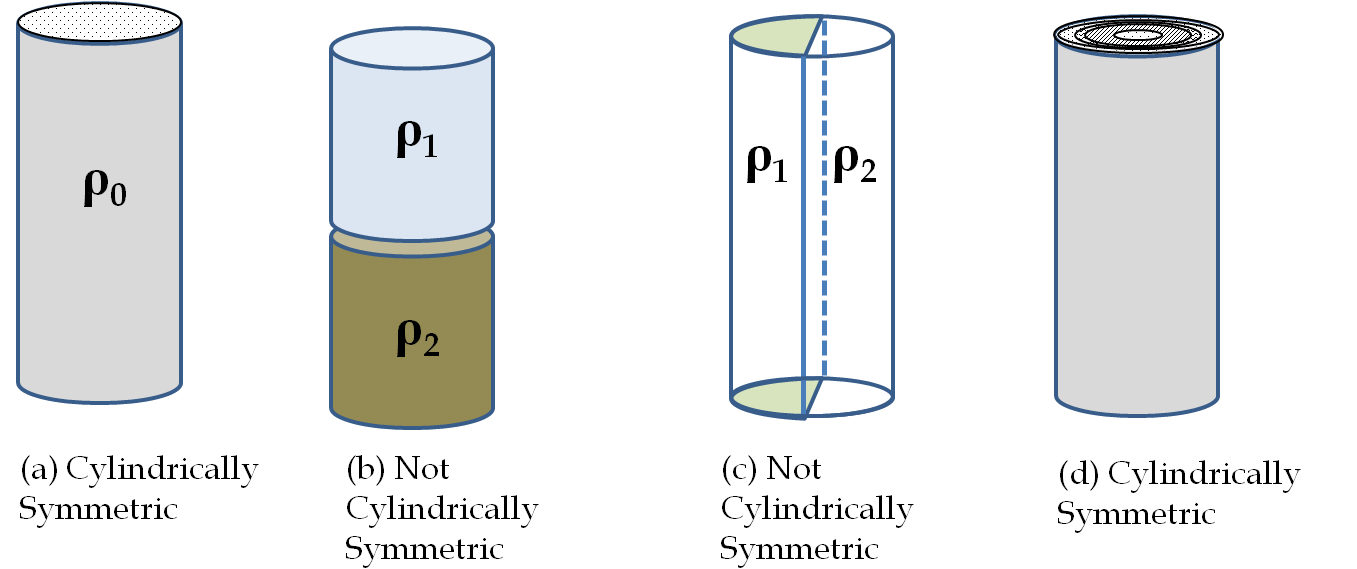Figure 30.4.1. Cylindrical symmetry. To determine if a given charge distribution has a cylindrical symmetry, you look at the cross-section of an infinitely long cylinder. If the charge density does not depend on the polar angle of the cross-section or along the axis, then you have a cylindrical symmetry. In (a) charge density is constant in the cylinder, in (b) upper half of the cylinder has a different charge density that the lower half, in (c) left half of the cylinder has a different charge density than the right half, and in (d) charges are constant in different cylindrical rings but the density does not depend on the polar angle. Cases (a) and (d) have cylindrical symmetry, and, (b) and (c) do not.

### Subsection30.4.2Consequences of Cylindrical Symmetry

When a charge distribution has cylindrical symmetry, there is no preferred direction in the cross-section plane of the cylinder and there is no dependence along axis. Therefore, the magnitude of the electric field of a cylindrical symmetric situation can only be a function of the distance from the axis of the cylinder.

The direction of electric field must be perpendicular to the axis. We can see this easily from the way we found electric field of a charged wire in the last chapter. Every charge has a pairing charge in the cylinder that will cancel components of the electric field that are not perpendicular to the axis of the cylinder.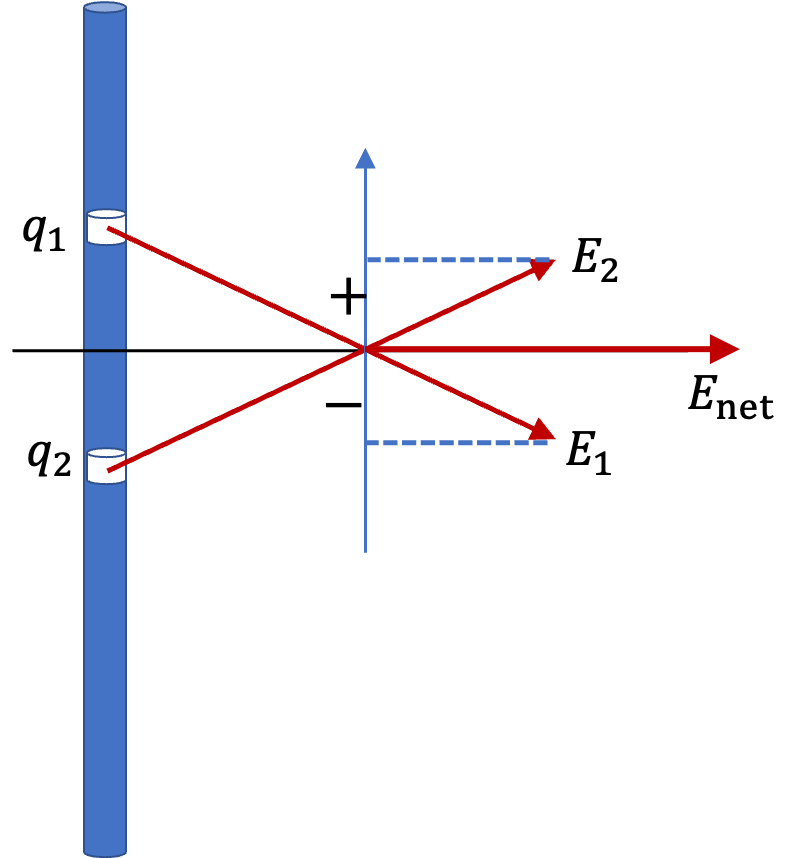These observations about the expected electric field are best cast in the cylindrical coordinate system illustrated in Figure 30.4.2. We use $z$ for the axis and polar coordinates $(s,\ \phi)$ for the radial and azimuthal angles in the $xy$-plane. We use letter $s$ rather than $r$ for the radial distance, since we would reserve $r$ for spherical radial distance, not radial distance in the $xy$-plane.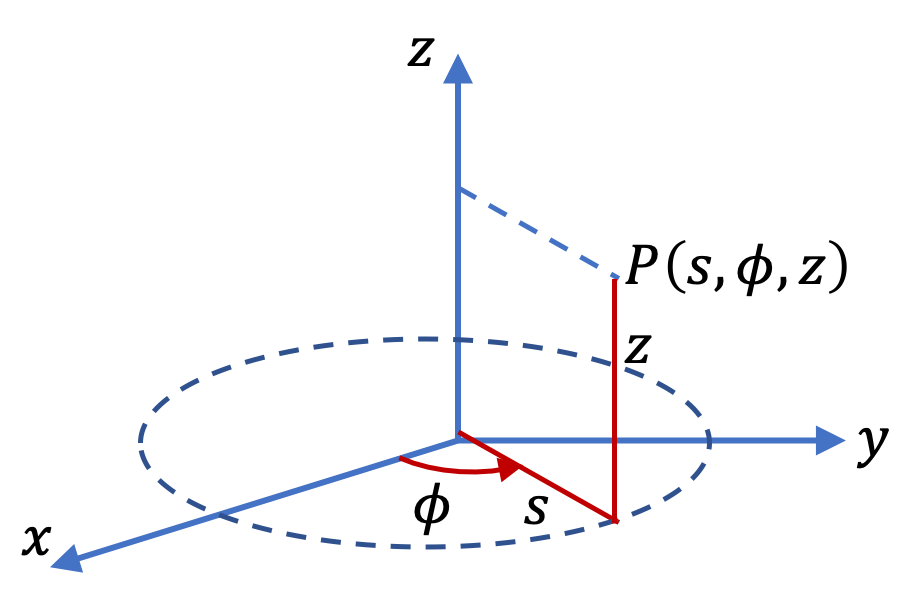Figure 30.4.2. The cylindrical coordinates consist of polar coordinates $(s,\ \phi)$ in the cross-section plane of the cylinder and a Cartesian axis $(z)$ along the axis of the cylinder.

Using cylindrical coordinates, we can assert that in case of cylindrical symmetry, the magnitude of electric field at a point will a function on $s$ only.

\begin{equation*} E_P = E_P(s), \end{equation*}

and the direction will be along the radial line to the axis, either away from the axis or towards the axis, depending upon the net positive or negative charge. We can include the direction information if we use a unit vector pointed away from the axis. We denote this unit vector by $\hat u_s\text{.}$ Then, electric field at P in vector form will be

\begin{equation} \vec E_P = E_P(s) \hat u_s.\tag{30.4.1} \end{equation}

### Subsection30.4.3Electric Field of a Uniformly Charged Cylinder

Consider a uniformly charged cylinder with volume charge density

\begin{equation*} \rho = \begin{cases} \rho_0 \amp 0\le s \le R\\ 0 \amp s \gt R. \end{cases} \end{equation*}

There are two types of points in this space, where we will find electric field.

1. a point $P_\text{out}$ outside the cylinder, $s \gt R\text{,}$ and
2. a point $P_\text{in}$ inside the sphere, $s \le R\text{.}$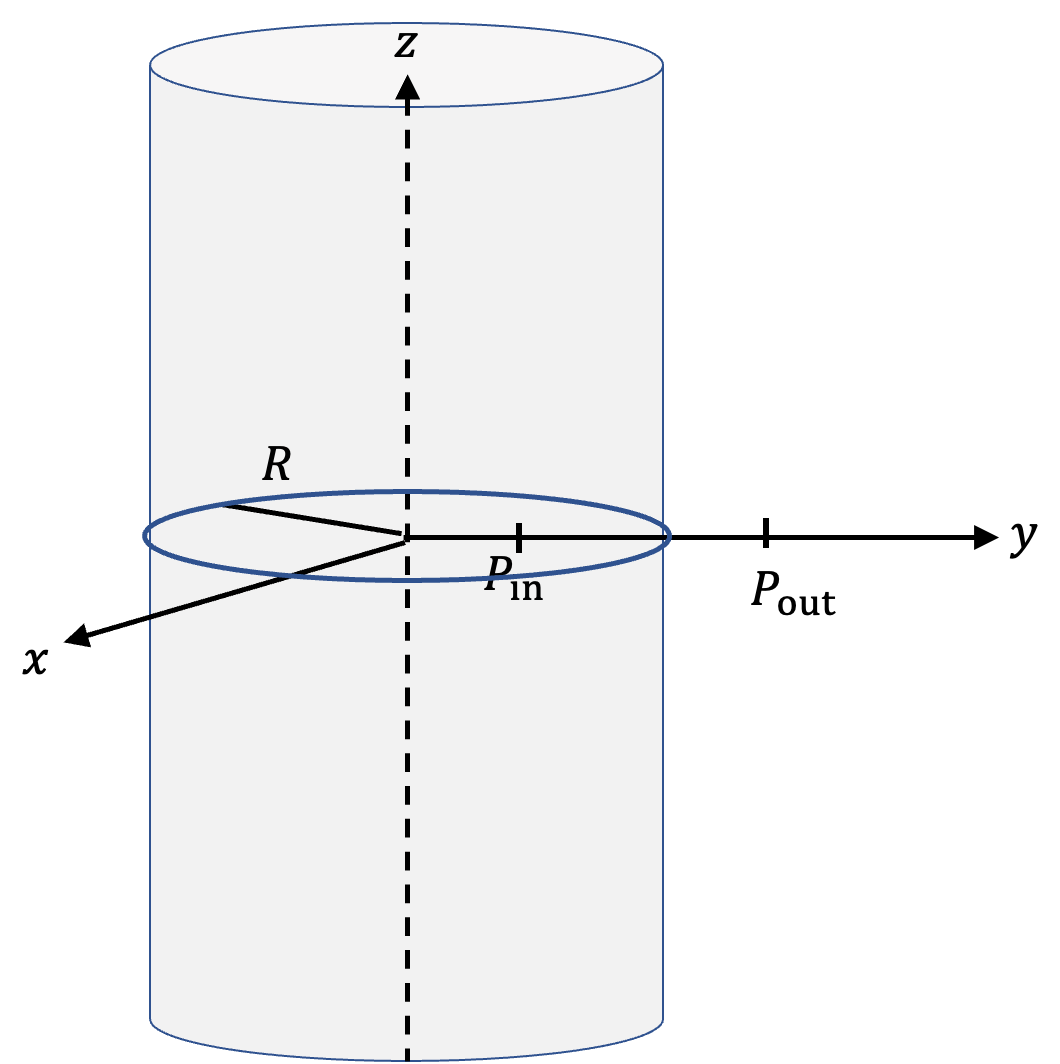The magnitude of electric field varies with distance by two different rules, one for points inside the sphere and another for point outside the sphere as we will derive below. This is also displayed in Figure 30.4.3.

\begin{equation*} E = \begin{cases} \dfrac{\rho_0}{2\epsilon_0}\, s\amp 0\le s \le R,\\ \dfrac{\rho_0}{2\epsilon_0}\, \dfrac{R^2}{s}\amp s \gt R. \end{cases} \end{equation*}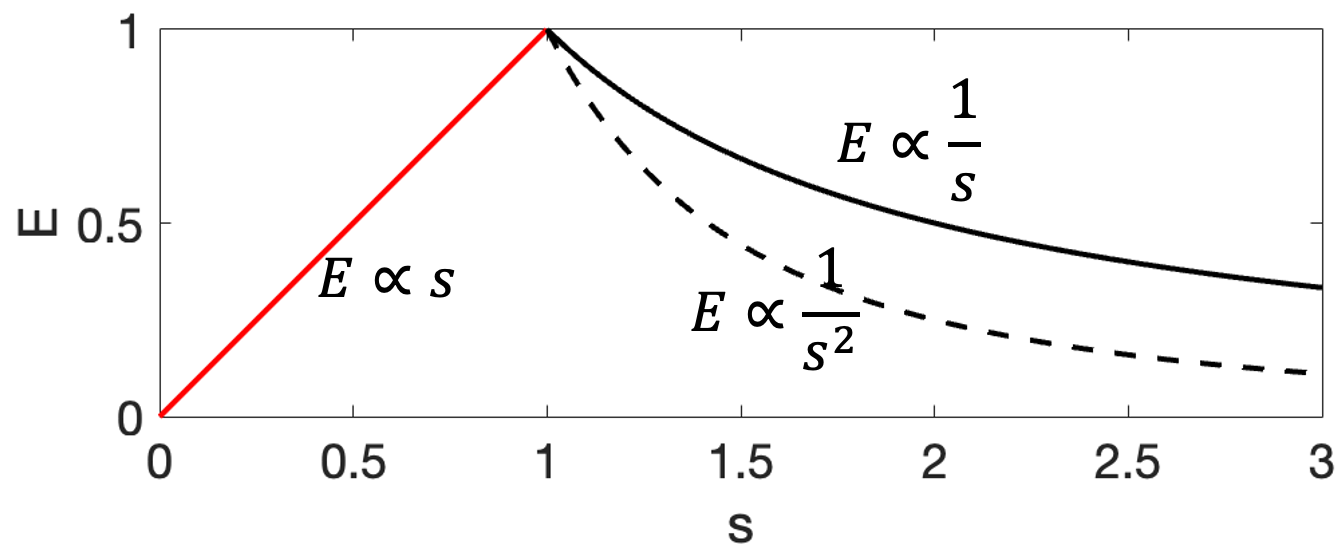Figure 30.4.3. Electric field of a uniformly charged non-conducting cylinder increases inside the cylinder to a maximum at the surface, and then decreases as $1/s\text{.}$ The dashed line shows $1/s^2$ for comparison of how rapidly electric field of a charged sphere drops with distance, which goes as $1/r^2\text{.}$ Here $s$ is in units of $R$ and $E$ has been scaled to have value $1$ at $s=1\text{.}$

The outside field is often written in terms of charge per unit length of the cylindrical charge. Multiplying $\rho_0$ by $\pi R^2$ will give charge per unit length of the cylinder. We denote this by $\lambda\text{.}$ Then, field outside the cylinder will be

\begin{equation*} E_\text{out} = \frac{\lambda}{2\pi\epsilon_0}\frac{1}{s}. \end{equation*}

#### Subsubsection30.4.3.1Deriving Electric Field at an Outside Point by Gauss's Law

Gaussian Surface and Flux

As before, I will call electric field at an outside point as $E_\text{out}\text{.}$ To show its functional dependence I will write the dependence on cylindrical radial distance $s$ explicitly.

\begin{equation*} E_\text{out} = E_\text{out}(s), \end{equation*}

Since in Gauss's law, electric field is inside an integral over a closed surface, we seek a Gaussian surface that contains point $P_\text{out}\text{,}$ where magnitude of electric field will not change over the surface. A cylindrical surface about the same axis is a good candidate to explore.

However, unlike the situation with spherical surface, a cylindrical surface has two types of surfaces as shown in Figure 30.4.4- (1) round surface at equal $s$ all around, and (2) the two flat ends, where $s$ goes from zero to the radius of the cylindrical surface.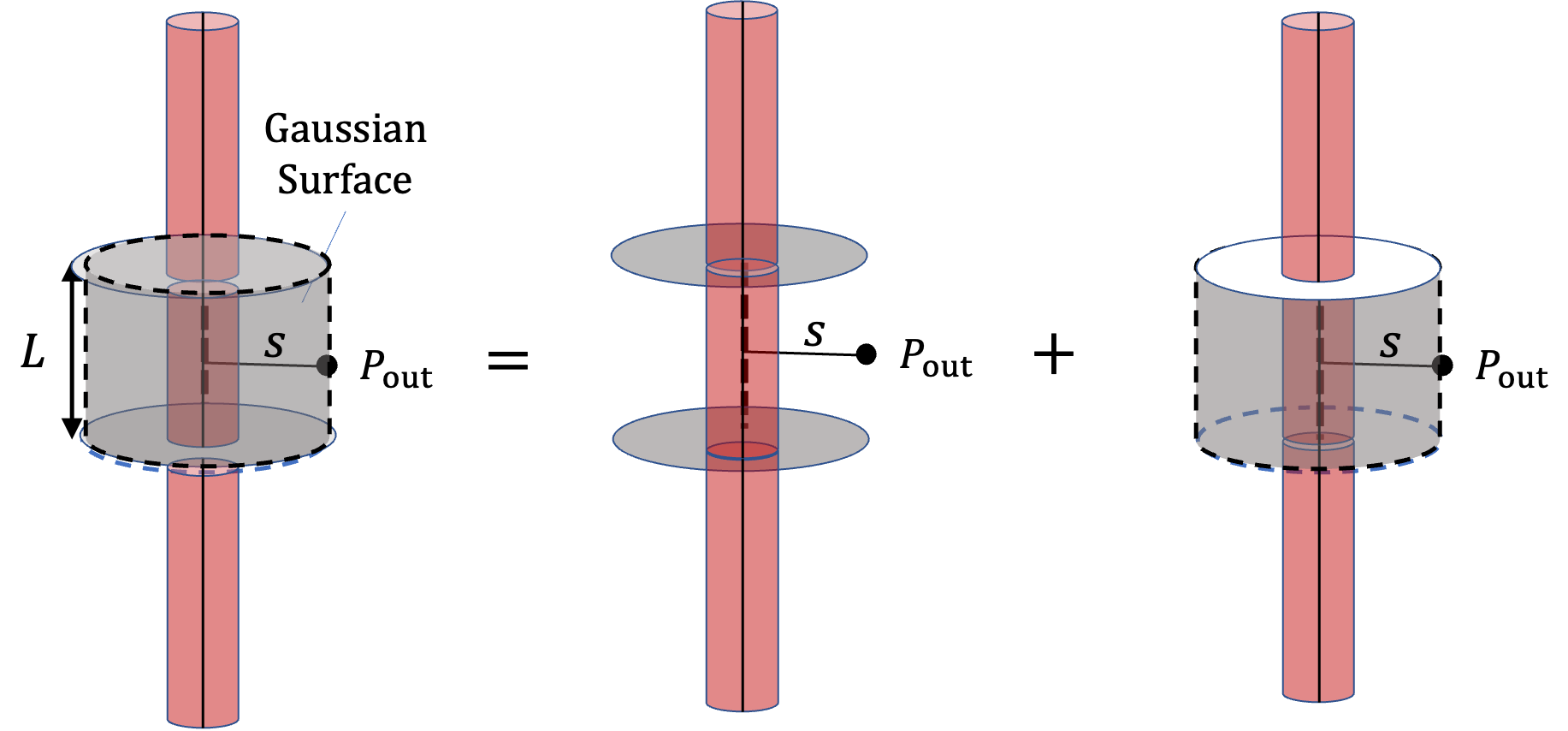Figure 30.4.4. The two types of surfaces make up the closed cylinderical Gaussian surface.

The flux in Gauss's law will be a sum of the fluxes on all of these surfaces combined. Fortuantely, the fluxes of the flat ends for cylindrical symmetry electric fields are zero due to the fact that direction of the electric field is along the surface and hence electric field lines do not pierce these surfaces. Another way to look at it is to note that dot product of the area vector and electric field is zero on these flat ends.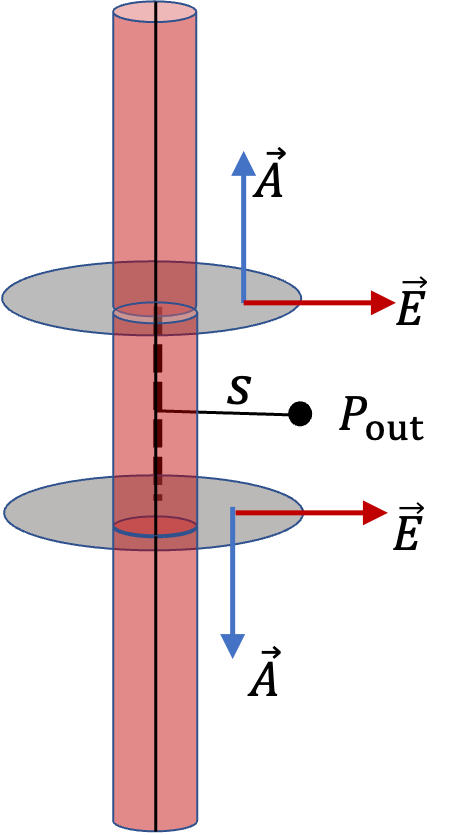The flux through the circular part of the cylinder is quite easy to work out since we magnitude of electric field is same at all points, which are denoting by $E_\text{out}(s)\text{,}$ and the direction of electric field at a patch is parallel to the area vector. Using the dot product form of flux, we get

\begin{equation*} \Phi_\text{round part} = E_\text{out}(s)\times 2\pi s L. \end{equation*}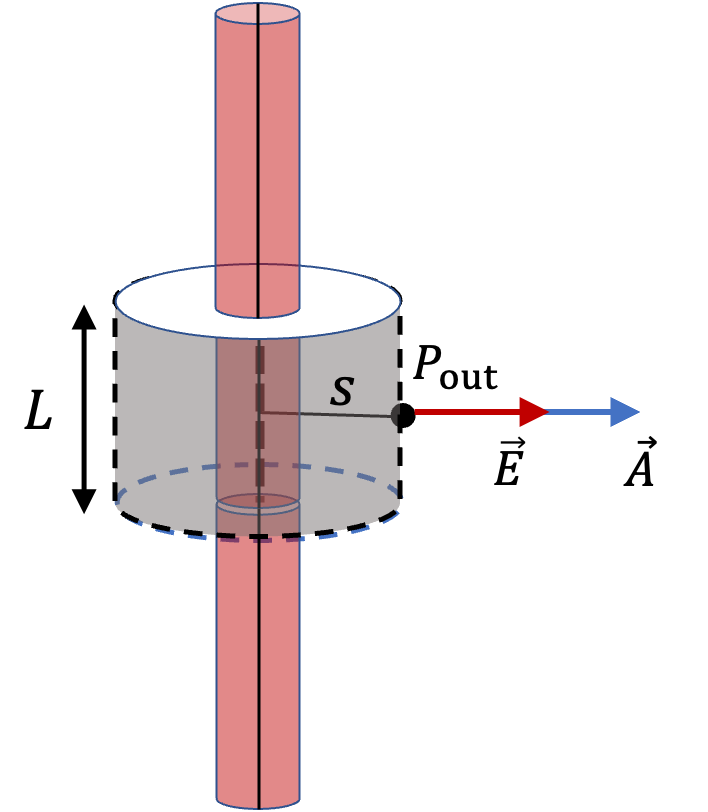Note that $L$ is the height of the Gaussian cylinder, not that of the charged cylinder, which is infinitely long.

Adding up the fluxes from the round part and the at the flat ends, we get the net flux through a closed surface to be

\begin{equation} \Phi_\text{closed surface} = E_\text{out}(s)\times 2\pi s L.\label{eq-gauss-cylinder-outside-flux}\tag{30.4.2} \end{equation}

Enclosed Charge:

Now, we find amount of charge enclosed by the closed surface. We notice that only a length $L$ of the charged cylinder is enclosed. Since charge density is constant here, corresponding charge is just the product of charge density and volume.

\begin{equation} q_\text{enc} = \rho_0 \times \pi R^2 L.\label{eq-gauss-cylinder-outside-enclosed-charge}\tag{30.4.3} \end{equation}

Use Gauss's Law:

Now, we use Gauss's law on flux in Eq. (30.4.2) and enclosed charge in (30.4.3). to get

\begin{equation*} E_\text{out}(s)\times 2\pi s L = \frac{\rho_0 \times \pi R^2 L}{\epsilon_0}. \end{equation*}

From this, we get the magnitude of electric field to be

\begin{equation} E_\text{out}(s) = \frac{\rho_0}{2\epsilon_0} \frac{R^2}{s}.\tag{30.4.4} \end{equation}

#### Subsubsection30.4.3.2Deriving Electric Field at an Inside Point by Gauss's Law

To derive the field at an inside point, we take a Gaussian cylindrical surface whose circular surface contains the field point of interest, i.e., point $P_\text{in}\text{.}$ We need to work out flux and enclosed charge here as well. The flux calculation is identical to the calculation given in Eq. (30.4.2) above for $P_\text{out}\text{.}$

\begin{equation*} \Phi_\text{closed surface} = E_\text{in}(s)\times 2\pi s L. \end{equation*}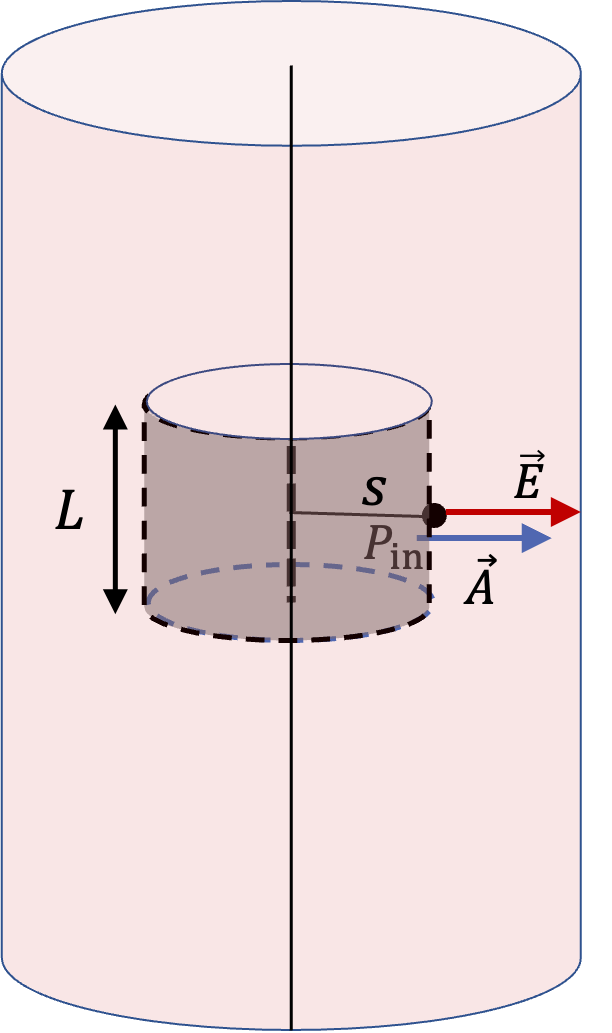For enclosed charge, we note here that, not all charges of the cylinder of length $L$ are enclosed. Only charges upto the radius of $s$ are enclosed. Therefore,

\begin{equation*} q_\text{enc} = \rho_0 \times \pi s^2 L. \end{equation*}

Now, we use them in Gauss's law to get

\begin{equation*} E_\text{in}(s)\times 2\pi s L = \frac{\rho_0 \times \pi s^2 L}{\epsilon_0}. \end{equation*}

Solving this for $E_\text{in}(s)$ we get

\begin{equation} E_\text{in}(s) = \frac{\rho_0}{2\epsilon_0}\ s.\tag{30.4.5} \end{equation}

Determine if approximate cylindrical symmetry holds for the following situations. State why or why not.

1. A $300$-cm long copper rod of radius $1$ cm is charged with $+500$ nC of charge and we seek electric field at a point $5$ cm from the center of the rod.
2. A $10$-cm long copper rod of radius $1$ cm is charged with $+500$ nC of charge and we seek electric field at a point $5$ cm from the center of the rod.
3. A $150$-cm wooden rod is glued to a $150$-cm plastic rod to make a $300$-cm long rod, which is then painted with a charged paint so that one obtains a uniform charge density. The radius of each rod is $1$ cm, and we seek an electric field at a point that is $4$ cm from the center of the rod.
4. Same rod as (c), but we seek electric field at a point that is $500$-cm from the center of the rod.
Hint

See solution.

(a) Yes, (b) No, (c) Yes, (c) No.

Solution 1 (a)

(a) Yes, approximate cylindrical symmetry exists, since the distance 5 cm $\lt\lt$ length of the rod 300 cm.

Solution 2 (b)

(b) No, cylindrical symmetry is not appropriate here, since distance to the space point, 5 cm is not much smaller than the size of the cylinder 10 cm.

Solution 3 (c)

(c) Although we have different materials, but since the charge density is uniform, the difference in material will not matter. The ends of the rod are far away, and hence cylindrical symmetry can be used in this case.

Solution 4 (d)

(d) Now, the cylindrical symmetry will not be appropriate here since ends of the cylinder are not far away compared to the distance to the space point.

A thin straight wire has a uniform linear charge density $\lambda_0$ (SI units: $\text{C/m}$). Find the electric field at a distance $d$ from the wire.

Hint

Use cylindrical symmetry.

Magnitude: $E = \dfrac{1}{2\pi\epsilon_0}\, \dfrac{\lambda_0}{d}\text{,}$ and direction away from the wire if $\lambda_0\gt 0$ and towards the wire if $\lambda_0\lt 0\text{.}$

Solution

The given charge density has cylindrical symmetry. Therefore, electric field at a distance $d$ from the wire will have the magnitude

\begin{equation*} E = \dfrac{1}{2\pi\epsilon_0}\, \dfrac{q_\text{enc}/L}{d}, \end{equation*}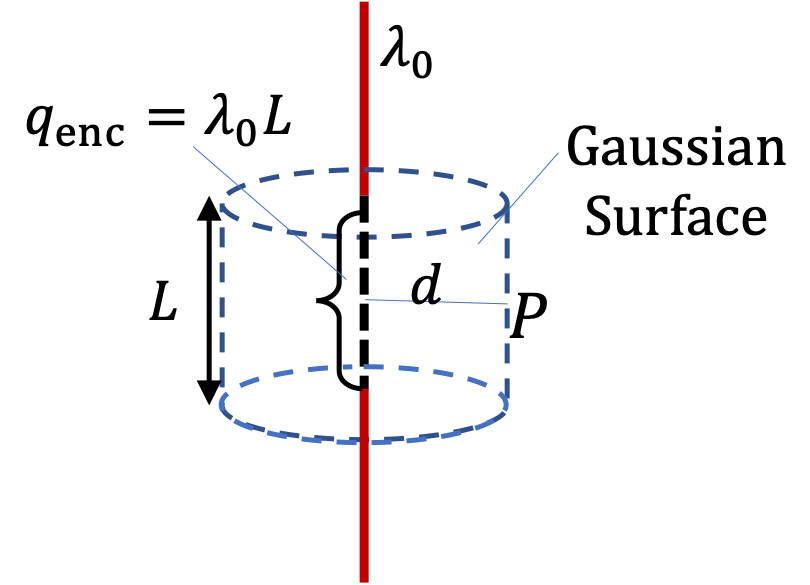where $q_\text{enc}$ are the charges on the wire in length $L\text{.}$

\begin{equation*} q_\text{enc} = \lambda_0 L. \end{equation*}

Therefore,

\begin{equation*} E = \dfrac{1}{2\pi\epsilon_0}\, \dfrac{\lambda_0}{d}. \end{equation*}

The direction of the field is away from the wire if $\lambda_0\gt 0$ and towards the wire if $\lambda_0\lt 0\text{.}$

A non-conducting cylindrical shell of radius $R$ has a uniform surface charge density $\sigma_0$ (SI units: $\text{C/m}^2$). Find the electric field (a) at a point outside the shell and (b) at a point inside the shell.

Hint

Use cylindrical symmetry.

(a) Magnitude $\frac{2\pi R \sigma_0}{2\pi \epsilon_0}\frac{1}{s}$ with direction away from axis if $\sigma_0 \gt 0$ and towards the axis if $\sigma_0 \lt 0\text{.}$ (b) $0\text{.}$

Solution 1 (a)

(a) Electric field at a point outside the shell

For a point outside the cylindrical shell, the Gaussian surface will be the surface of a cylinder of radius $s \gt R$ and length $L$ as shown in the figure.

The charge enclosed by the Gaussian cylinder is equal to the charge on the cylindrical shell of length L. Therefore, $\lambda_\text{enc}$ is given by

\begin{equation*} \lambda_\text{enc} = \frac{\sigma_0\times 2\pi R L}{L} = 2\pi R \sigma_0. \end{equation*}

Hence, the electric field at a point P outside the shell at a distance s away from the axis has the magnitude:

\begin{equation*} E_P = \frac{2\pi R \sigma_0}{2\pi \epsilon_0}\frac{1}{s} \ \ \ (s\gt R), \end{equation*}

The electric field at P will be pointed away from the axis as given in Figure 30.4.8 if $\sigma_0 \gt 0$ , but towards the axis if $\sigma_0 \lt 0\text{.}$

Solution 2 (b)

(b) Electric field at a point inside the shell

For a point inside the cylindrical shell, the Gaussian surface will be a cylinder whose radius $s$ is less than $R\text{.}$ That means, no charges will be included inside the Gaussian surface.

\begin{equation*} \lambda_{enc} = 0. \end{equation*}

This gives the following equation for the magnitude of the electric field $E_{in}$ at a point whose $s$ is less than $R$ of the shell of charges.

\begin{equation*} E_\text{in}\times 2\pi s L = 0\ \ \ (s\lt R), \end{equation*}

which gives

\begin{equation*} E_\text{in} = 0\ \ \ (s\lt R). \end{equation*}

Figure shows two charged concentric thin cylindrical shells. There are no charges in the space at the core, i.e., charge density, $\rho = 0,\ r\lt R_1$ or between the shells $\rho = 0,\ R_1\lt r \lt R_2\text{.}$ The two shells are uniformly charged with different charge densities, $+\sigma_1$ and $-\sigma_2$ such that the net charge on the two shells are equal in magnitude but opposite in sign.

There are three distinct field points, labeled, $P_1\text{,}$ $P_2\text{,}$ and $P_3\text{,}$ which are distances $s_1\text{,}$ $s_2\text{,}$ and $s_3$ from the axis. (a) Find electric fields at these points. (b) Draw representative electric field lines for this system of charges.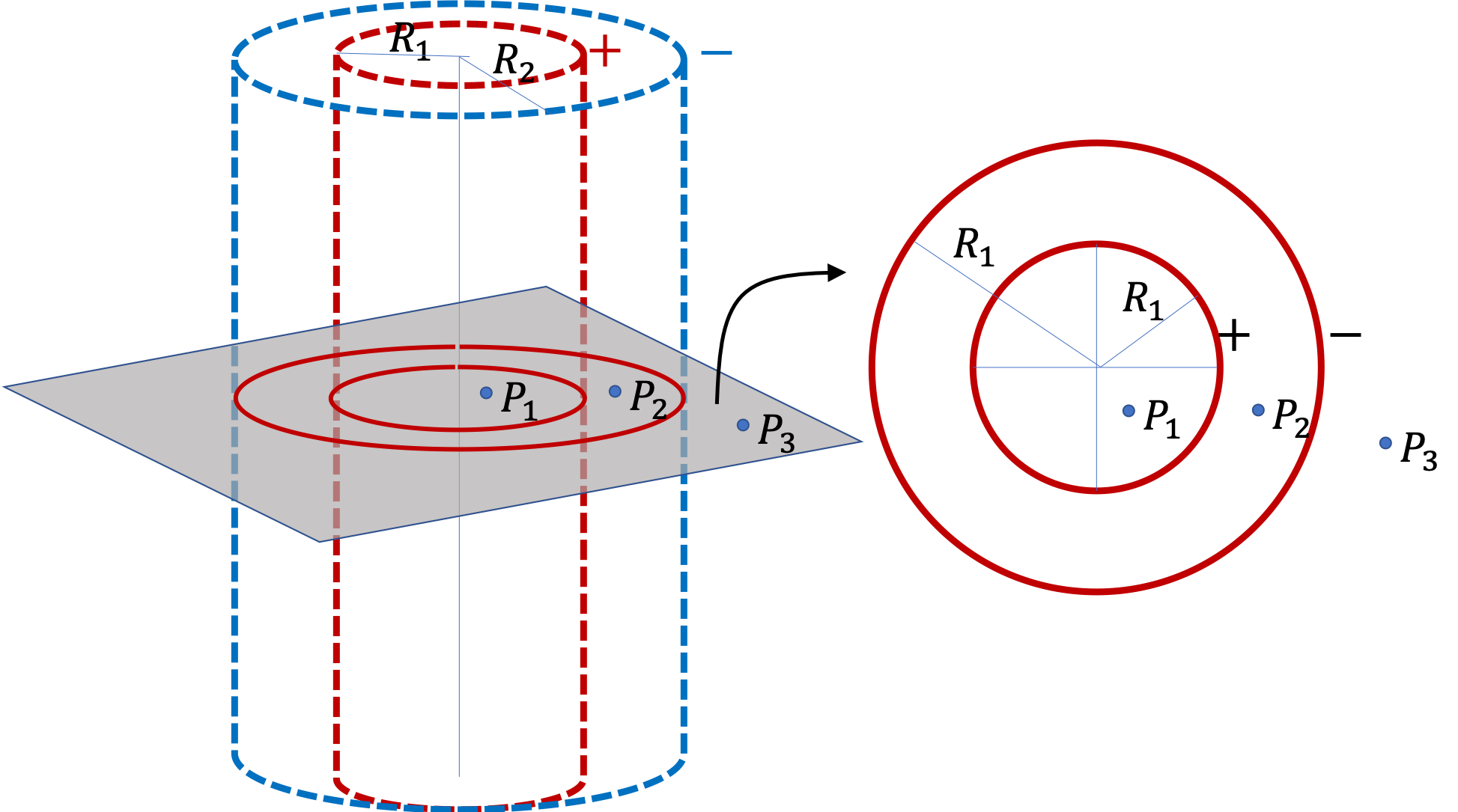How can you create this type of situation? You can start with two concentric metal cylindrical shells. Place some positive charge on inner shell and same amount on the outer shell. You can do that by connecting a positive terminal of a DC battery to the inner shell and the negative of the battery to the outer shell. This arrangement of metal shells is called a cylindrical capacitor. We will study capacitors in a future chapter.

Hint

Use cylindrical symmetry.

$E_\text{between} = \dfrac{\sigma_1 R_1}{\epsilon_0}\, \dfrac{1}{s_2}\text{.}$

Solution 1 (a)

The given charges satisfy the condition of cylindrical symmetry. Therefore, we use Gaussian cylinders with the field point of interest $(P_1,\ P_2, \text{ or } P_3)$ at the side of the cylinder. This will give smae formula for the magnitude of electric field at these points.

\begin{equation*} E_i = \dfrac{1}{2\pi\epsilon_0}\, \dfrac{\lambda_\text{inc,i}}{s_i}, \ \ (i=1,\ 2, \ 3), \end{equation*}

where $i$ refer to the three points of interest. The enclosed charges inside the Gaussian cylinders in the three cases give

\begin{align*} \lambda_\text{inc,1} \amp = 0,\\ \lambda_\text{inc,2} \amp = \dfrac{\sigma_1 \times 2\pi R_1 L}{L},\\ \lambda_\text{inc,3} \amp = \dfrac{\sigma_1 \times 2\pi R_1 L - \sigma_2 \times 2\pi R_2 L}{L} = 0. \end{align*}

Therefore, the magnitudes of electric fields at these points are

\begin{align*} E_1 \amp = 0,\\ E_2 \amp = \dfrac{\sigma_1 R_1}{\epsilon_0}\, \dfrac{1}{s_2},\\ E_3 \amp = 0. \end{align*}
Solution 2 (b)

In the present situaion, electric field is non-zero only between the shells with direction radially outward from the positive shell to the negative shell. It is better to draw these lines in a cross-section plane of the cylinder.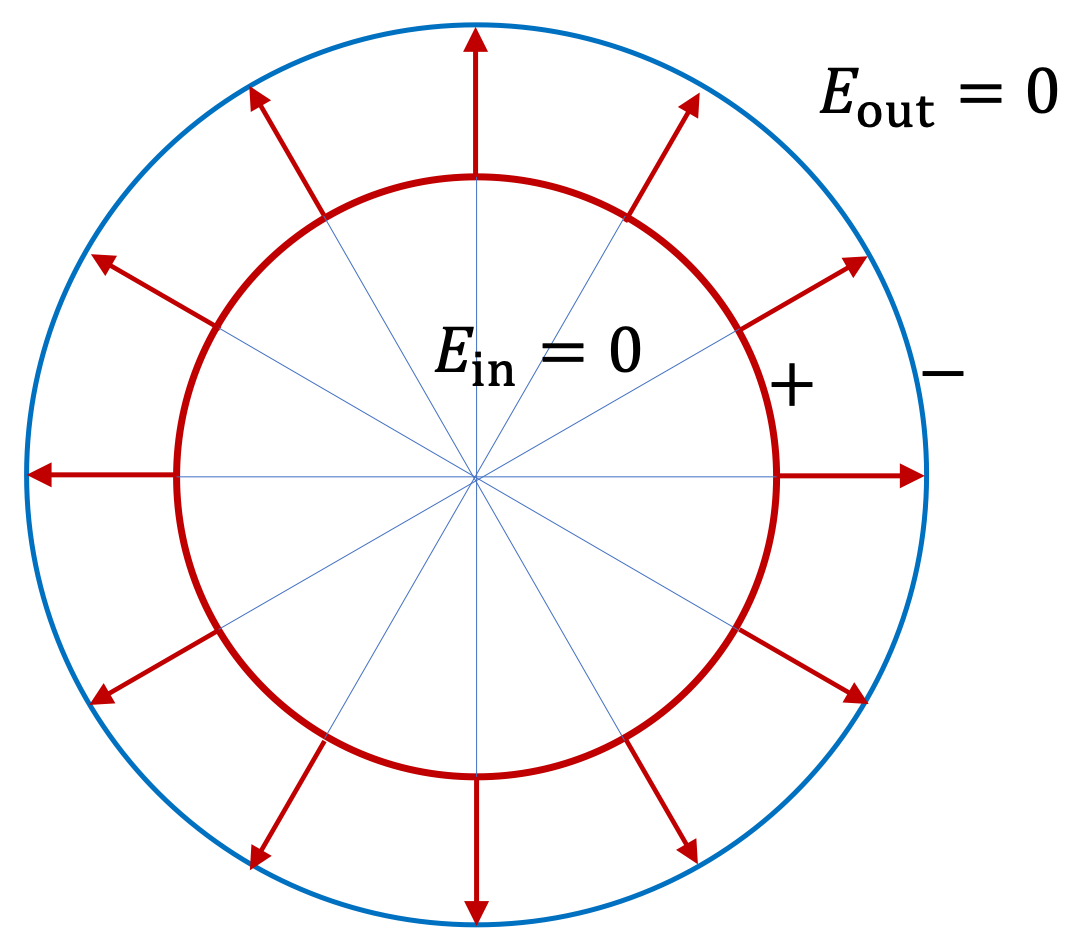A long rod of radius $R_1$ is uniformly charged with volume charge density $\rho_0\text{.}$ Surrounding the rod is a shell of radius $R_2$ that is also charged uniformly, but of the opposite type and has a surface charge density $-\sigma_0\text{.}$ The two charge densities are such that for any length the rod and the shell are balanced in total charges. Find electric field in (a) $s \le R_1\text{,}$ (b) $R_1 \lt s \lt R_2\text{,}$ (c) $s \gt R_2\text{.}$

Hint

Apply cylindrical symmetry.

(a) $\frac{\rho }{2\epsilon_0}\ s\text{,}$ (b) $\frac{\lambda_0}{2\pi \epsilon_0}\ \frac{1}{s}$ with $\lambda_0 = \rho_0 \pi R_1^2\text{,}$ (c) $0\text{.}$

Solution

(a) Assuming the rod and shell are long enough that we can assume cylindrical symmetry, we can use immediately use th results of Gauss's law in this section. For (a), only the charge in the inner cylinder matters and the case is that of an inside point. This gives

\begin{equation*} 2\pi s L E_a = \frac{\rho \pi s^2 L}{\epsilon_0}. \end{equation*}

Therefore,

\begin{equation*} E_a = \frac{\rho }{2\epsilon_0}\ s. \end{equation*}

(b) This point is an outside point of the inner cylinder, but inside a shell. Hence, only inside cylinder matters. This gives

\begin{equation*} E_b = \frac{\lambda_0}{2\pi \epsilon_0}\ \frac{1}{s}, \end{equation*}

where $\lambda_0 = \rho_0 \pi R_1^2\text{.}$

(c) Here, Gauss's equation for a Gaussian surrounding both cylinder and shell will give

\begin{equation*} 2\pi s L E_c = 0. \end{equation*}

Therefore, $E_c=0\text{.}$